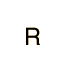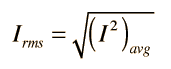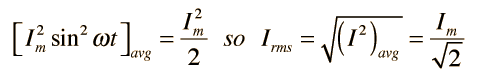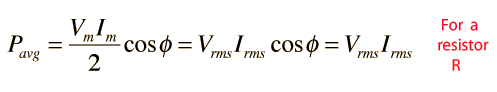# Resistor AC Response

 ImpedanceCalculateExamine Capacitor InductorContribution tocomplex impedance Phasor diagramFor ordinary currents and frequencies the behavior of a resistor is that of a dissipative element which converts electrical energy into heat. It is independent of the direction of current flow and independent of the frequency. So we say that the AC impedance of a resistor is the same as its DC resistance. That assumes, however, that you are using the rms or effective values for the current and voltage in the AC case.

Index

AC Circuits

 HyperPhysics***** Electricity and Magnetism R Nave
Go Back

# RMS and Effective Values

Circuit currents and voltages in AC circuits are generally stated as root-mean-square or rms values rather than by quoting the maximum values. The root-mean-square for a current is defined byThat is, you take the square of the current and average it, then take the square root. When this process is carried out for a sinusoidal currentSince the AC voltage is also sinusoidal, the form of the rms voltage is the same. These rms values are just the effective value needed in the expression for average power to put the AC power in the same form as the expression for DC power in a resistor. In a resistor where the power factor is equal to 1:Since the voltage and current are both sinusoidal, the power expression can be expressed in terms of the squares of sine or cosine functions, and the average of a sine or cosine squared over a whole period is = 1/2.

 Average of trig functions
Index

AC Circuits

 HyperPhysics***** Electricity and Magnetism R Nave
Go Back